# Proton 2: 2nd Degree (P2-20)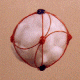The Proton Shell of 2 is built from six hadron strings. Two of the strings are equatorial, passing through four quarks, the other four strings each pass through three of the quarks. The number of quarks a string passes through is equal to the number of snap points for the string, and must be at least 3 for a nuclear string (one which lies on the nuclear surface).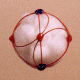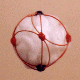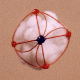## Stereograms of N2-20 and P2-20 Shell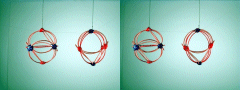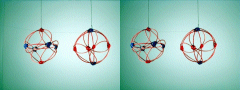The left model in each image is the N2-20 shell, the right is the P2-20. These shells will stack as a complementary pair forming Helium 4. I suspect the proton shell may be able to take the inner shell position in He-4, and the electron pair may be harbored in the nuclear interior, preventing helium-4 from forming electron-mediated bonds, thus making it inert.

 Required Snap Points: 20 Available Snap Points: 20 Pinned Vector Bosons: 0 L1 = 19.36959362" (equatorial) L2 = 15.81520319" (filler) Model Diameter: 6.165533141" Loop Ratio (1:2): 1.224745164 Loop Equation: 2(L1) + 4(L2) = 102" Snap Point Equation: 2(4) + 4(3) = 20 R.S.P.

Copyright 1997 by Arnold J. Barzydlo
Go Back